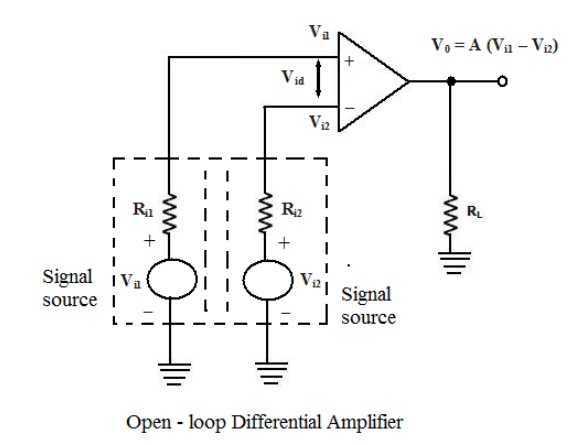Home | | Linear Integrated Circuits | Open loop-op-amp Configuration

# Open loop-op-amp Configuration

There are three open – loop configurations of op-amp namely 1. differential amplifier 2. Inverting amplifier 3. Non-inverting amplifier

Open loop-op-amp Configuration:

The term open-loop indicates that no feedback in any form is fed to the input from the output. When connected in open – loop, the op-amp functions as a very high gain amplifier. There are three open – loop configurations of op-amp namely

1.        differential amplifier

2.        Inverting amplifier

3.        Non-inverting amplifier

The above classification is made based on the number of inputs used and the terminal to which the input is applied. The op-amp amplifies both ac and dc input signals. Thus, the input signals can be either ac or dc voltage.

1. Open–loop Differential Amplifier:

In this configuration, the inputs are applied to both the inverting and the non-inverting input terminals of the op-amp and it amplifies the difference between the two input voltages. Figure shows the open-loop differential amplifier configuration.

The input voltages are represented by Vi1 and Vi2. The source resistance Ri1 and Ri2 are negligibly small in comparison with the very high input resistance offered by the op-amp, and thus the voltage drop across these source resistances is assumed to be zero. The output voltage V0 is given by

V0 = A(Vi1 – Vi2 )

where A is the large signal voltage gain. Thus the output voltage is equal to the voltage gain A times the difference between the two input voltages. This is the reason why this configuration is called a differential amplifier. In open – loop configurations, the large signal voltage gain A is also called open-loop gain A.2. Inverting amplifier:In this configuration the input signal is applied to the inverting input terminal of the op-amp and the non-inverting input terminal is connected to the ground. Figure shows the circuit of an open – loop inverting amplifier.

The output voltage is 1800 out of phase with respect to the input and hence, the output voltage V0 is given by,

V0 = -AVi

Thus, in an inverting amplifier, the input signal is amplified by the open-loop gain A and in phase

– shifted by 1800.

3. Non-inverting AmplifierFigure shows the open – loop non- inverting amplifier. The input signal is applied to the non-inverting input terminal of the op-amp and the inverting input terminal is connected to the ground.

The input signal is amplified by the open – loop gain A and the output is in-phase with input signal.

In all the above open-loop configurations, only very small values of input voltages can be applied. Even for voltages levels slightly greater than zero, the output is driven into saturation, which is observed from the ideal transfer characteristics of op-amp shown in figure. Thus, when operated in the open-loop configuration, the output of the op-amp is either in negative or positive saturation, or switches between positive and negative saturation levels. This prevents the use of open – loop configuration of op-amps in linear applications.

Limitations of Open – loop Op – amp configuration:

Firstly, in the open – loop configurations, clipping of the output waveform can occur when the output voltage exceeds the saturation level of op-amp. This is due to the very high open – loop gain of the op-amp. This feature actually makes it possible to amplify very low frequency signal of the order of microvolt or even less, and the amplification can be achieved accurately without any distortion. However, signals of such magnitudes are susceptible to noise and the amplification for those application is almost impossible to obtain in the laboratory.

Secondly, the open – loop gain of the op – amp is not a constant and it varies with changing temperature and variations in power supply. Also, the bandwidth of most of the open- loop op amps is negligibly small. This makes the open – loop configuration of op-amp unsuitable for ac applications. The open – loop bandwidth of the widely used 741 IC is approximately 5Hz. But in almost all ac applications, the bandwidth requirement is much larger than this.

For the reason stated, the open – loop op-amp is generally not used in linear applications. However, the open – loop op amp configurations find use in certain non – linear applications such as comparators, square wave generators and astablemultivibrators.

Study Material, Lecturing Notes, Assignment, Reference, Wiki description explanation, brief detail
Linear Integrated Ciruits : Characteristics of op-amp : Open loop-op-amp Configuration |